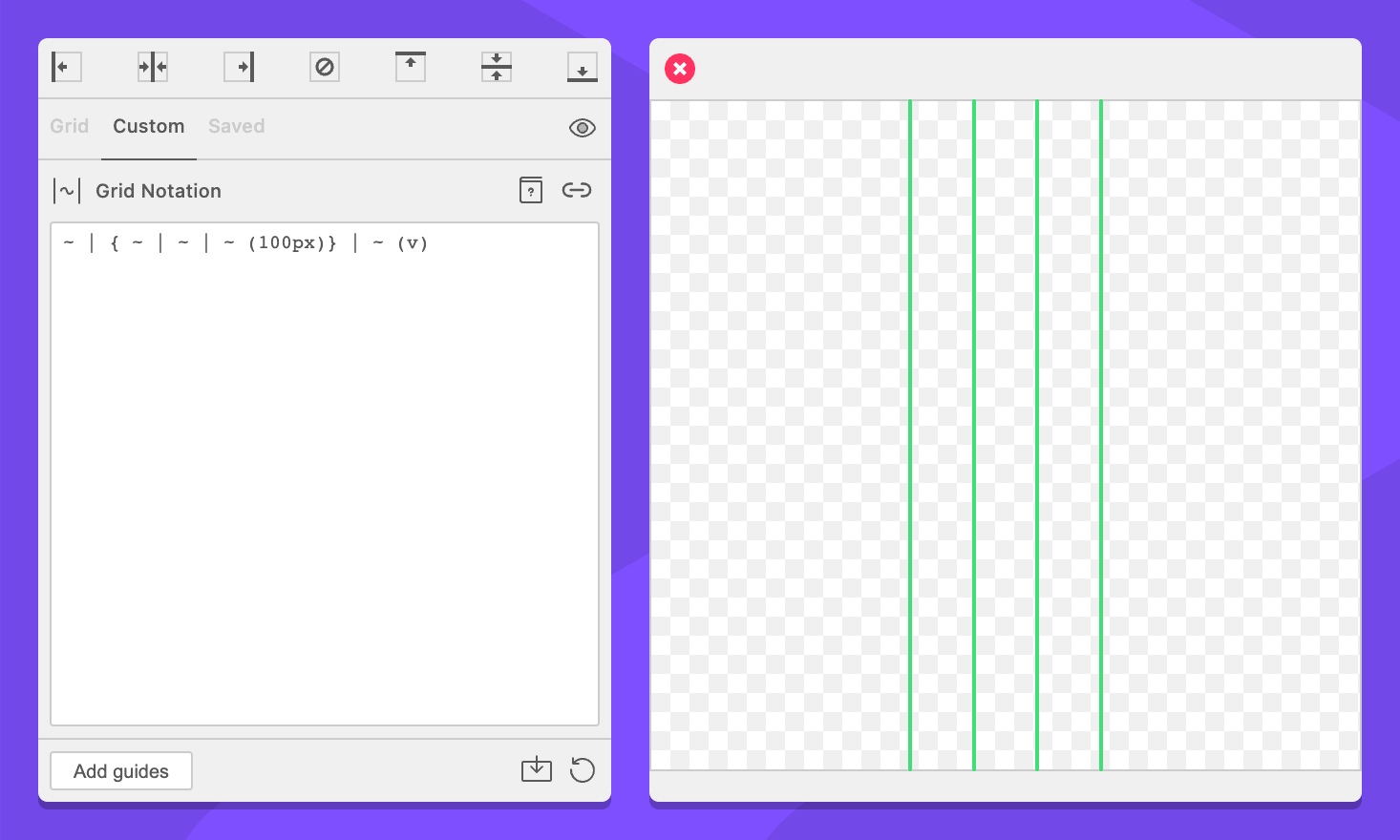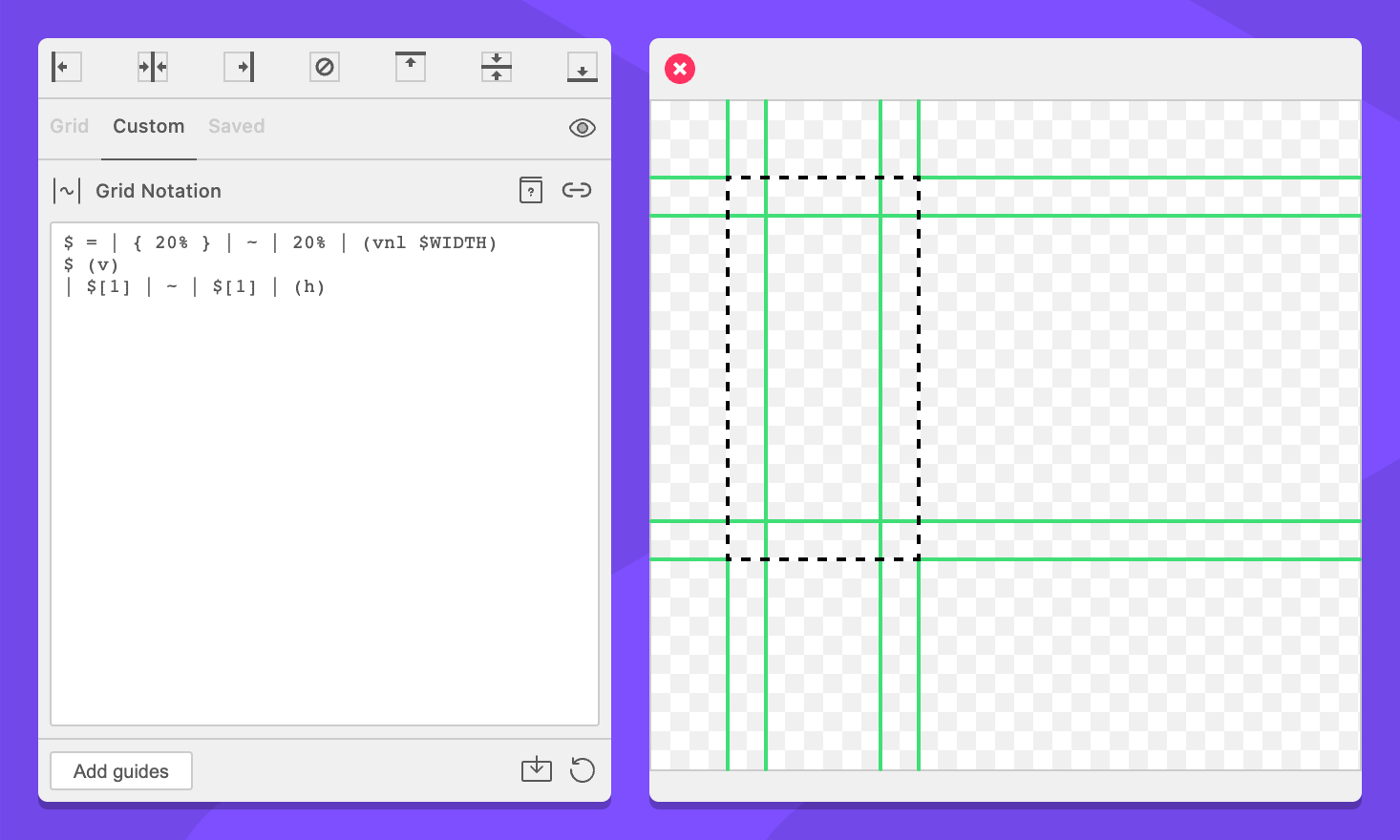# Blocks

Format: `{ <commands> }`
In words: “All of these commands are together”

Blocks allows you to isolate commands within a grid. A complete grid may be specified within the brackets of a block, including options.

This can be useful to change the context by which wildcards or percent units are calculated.

For example, The following example creates a centered three column grid.

``~ | { ~ | ~ | ~ (100px)} | ~ (v)``The wildcards within the block are calculated based on the 100px size, while the wildcards outside the block are calculated based on the size of the context minus the size of the block.

## Capturing Blocks

Format: `\$[<index>]`
In words: “Get the commands of the block at the index provided”

When block appear within a variable, their commands can be referenced later. Index 0 represents all commands within the variable. Each index after that corresponds to a capturing block within the variable.

``\$ = | { 10px } |``

In the the example above, `\$` would be `| 10px |` and `\$` would be `10px`.

When combined with variable flattening and calculated commands like wildcards and percent units, this enables us to to make references between grids. For example, a grid where all margins are a percentage of the total width of a space.

``````\$ = | { 20% } | ~ | 20% | (vnl \$WIDTH)

# Add our grid with vertical guides
\$ (v)

# Now do horizontal guides, referencing the margin from the vertical guides.
| \$ | ~ | \$ | (h)``````We can break that down into more understandable bits.

``\$ = | { 20% } | ~ | 20% | (vnl \$WIDTH)``

First we create a variable that holds our vertical guides. Our margins are each `20%` of grid, and the wildcard pushes them to the edges. We reference the special variable `\$WIDTH` as the size of the variable which forces grid notation to calculate the value of the percentage commands.

Importantly, we wrap one of the percent commands (it doesn’t matter which one) in a block so we’ll have access to it later. The command is calculated at this point, so we know that `\$` will be equal to our margin size.

``````# Add our grid with vertical guides
\$ (v)``````

Nothing too interesting here. We reference our variable and tell it to add our vertical guides.

``````# Now do horizontal guides, referencing the margin from the vertical guides.
| \$ | ~ | \$ | (h)``````

As mentioned before, we reference `\$` which is our calculated margin size, but this time we use horizontal guides.

## Limitations

• Blocks ignore the orientation setting. The enclosing grid determines the orientation of guides.